# Solving, Writing & Graphing Linear Equations Chapter Exam

Exam Instructions:

Choose your answers to the questions and click 'Next' to see the next set of questions. You can skip questions if you would like and come back to them later with the yellow "Go To First Skipped Question" button. When you have completed the practice exam, a green submit button will appear. Click it to see your results. Good luck!

### Page 2

#### Question 10 10. If the graph below has the equation Ax + By = -4, what must be true about A and B?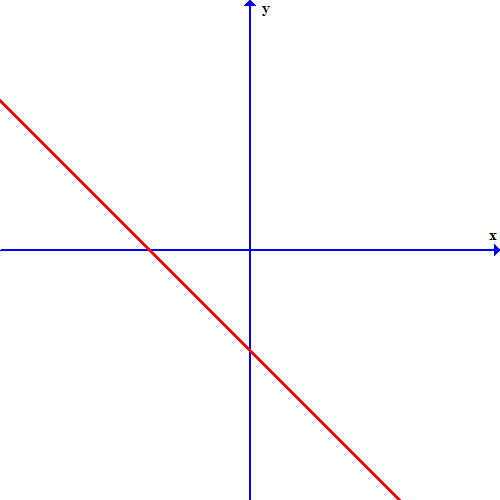### Page 3

#### Question 11 11. If the graph below was written in the slope intercept form, which of the following would be true?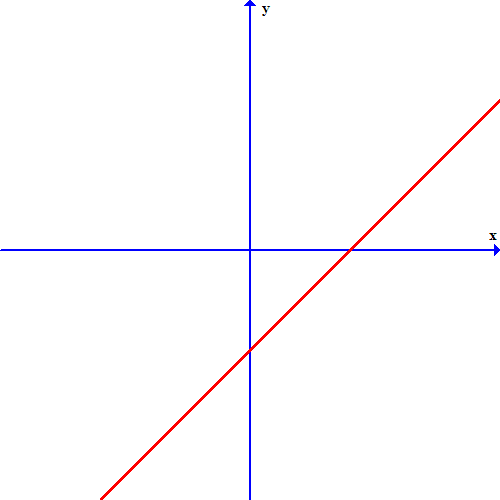#### Question 12 12. What is the slope-intercept form equation of the graph below?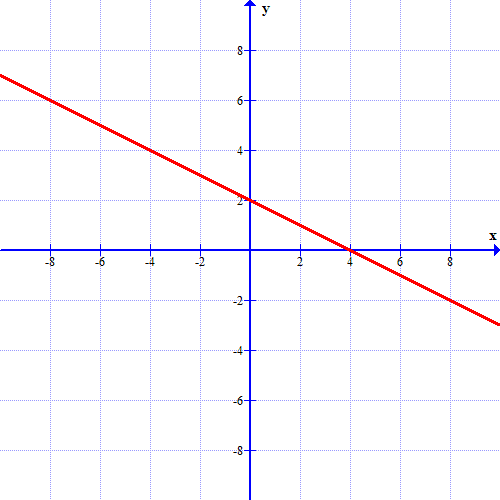#### Question 15 15. What is the equation of the graph below?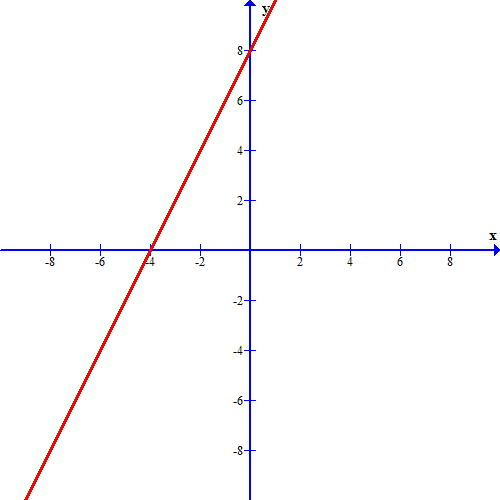### Page 4

#### Question 17 17. Which of these equations represents a line that is perpendicular to the line in the graph below?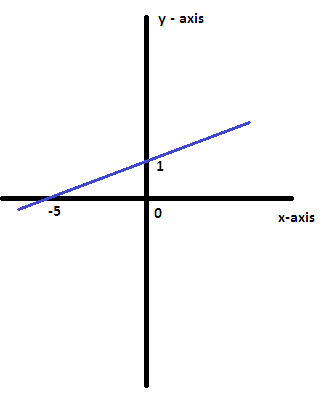### Page 5

#### Question 23 23. The equation for the following graph could be _____.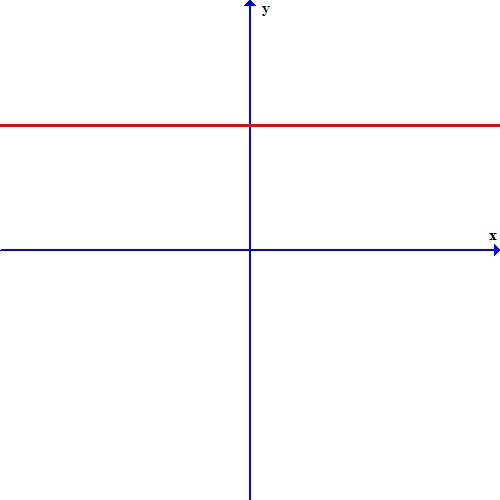### Page 6

#### Solving, Writing & Graphing Linear Equations Chapter Exam Instructions

Choose your answers to the questions and click 'Next' to see the next set of questions. You can skip questions if you would like and come back to them later with the yellow "Go To First Skipped Question" button. When you have completed the practice exam, a green submit button will appear. Click it to see your results. Good luck!

Support